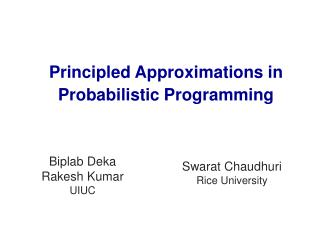DownloadDownload PresentationPrincipled Approximations in Probabilistic Programming

# Principled Approximations in Probabilistic Programming

Download Presentation## Principled Approximations in Probabilistic Programming

- - - - - - - - - - - - - - - - - - - - - - - - - - - E N D - - - - - - - - - - - - - - - - - - - - - - - - - - -
##### Presentation Transcript

1. Principled Approximations in Probabilistic Programming

2. The computing stack (approximation) Algorithms Compiler and runtime Architecture The APPROX view: with probabilitiesand approximations!

3. The computing stack Algorithms Compiler and runtime Architecture Probabilistic program Program synthesis Hardware for sampling The APPROX view: with probabilitiesand approximations!

4. DPMM Clustering Model • Gaussian Dirichlet Process Mixture Model (DPMM) Cluster 2 New Cluster Gibbs Sampling Algorithm Cluster 3 Cluster 2 Cluster 2 • In each iteration, update the cluster assignments of data points one at a time • For each data point, compute the probability of it belonging to all existing clusters and an unseen cluster • Sample from this probability distribution Cluster 1 Cluster 1 Cluster 1

5. Sampling in Hardware 35b 1b 32b Comparators Prefix Sum Encoder Probabilities 3b Sample 32b PRNG CLK Idea: exploit errors!

6. Robustness to hardware faults Transient Faults Stuck-at Faults Sampler Clustering using DPMM Deka, Biplab. “On Fault Tolerance of Hardware Samplers”. Masters Thesis, University of Illinois at Urbana Champaign, 2014

7. Approximating compilers Traditional compiler: • Input: Deterministic program • Goal: Executable semantically equivalent to source • Method: Syntax-guided translation Approximating compiler: • Input: Probabilistic program • Goal: • Satisfy basic boolean invariants • Minimize quantitative error • Method:Program synthesis

8. Probabilistic programs heightMan = Gaussian(177,64); heightWoman = Gaussian(164,64); assume(heightWoman > heightMan); return heightMan More complex example: clustering Addition: assertions, angelic nondeterminism Source: Tutorial on Infer.NET by John Winn and Tom Minka

9. Probabilistic programming ++ • Random variables X: range over distributions • Deterministic variables y • Either holesor temporaries • Functions f(X1,..., Xk, y1,..., yk) • Can map random variables to deterministic ones • Expectation, probability • Assertions • Pareto-optimality goals

10. Example X = Gaussian(??, 10); assume (X < 10); c = Pr(X > 0); assert (c > 0.7); minimize (c); Hole One synthesis algorithm in [CCS14] Based on probabilistic abstract interpretation [CCS14] Chaudhuri, Clochard, Solar-Lezama. Bridging boolean and quantitative synthesis using smoothed proof search. POPL 2014.

11. Use in approximation Holes = degree of approximation Assertions = invariants, hard bounds Declarative error minimization Deterministic temporaries track resource consumption Synthesis = Compilation

12. Thank you!Questions?